you are here:/ News & Insights / Engineering Advantage Blog / Incorporating the Stress Triaxiality Factor into FEA Simulations

# Incorporating the Stress Triaxiality Factor into FEA SimulationsApril 11, 2017 By: Eric Stamper

A common approach to assess the structural integrity of a design constructed from a ductile metal is to compare the Von-Mises stress, σVM, to the yield stress of the material. It’s also common for the yield stress to be determined from a uniaxial test, and to assume that this limit can also be used to evaluate a triaxial stress state, as would typically be experienced in a complex geometry. However, if the material yield stress in a pure shear condition, as in a torsional test, was determined, it would differ from the uniaxial yield stress from a tension test. Additionally, a review of three different stress-strain curves obtained from a tension, compression and torsion test, in Figure 1, above, shows that the shapes of the von Mises stress-strain curves, as well as the fracture strain, is based on the state of stress.

The premise that different states of stress will cause varying plasticity and failure, leads to defining specific constitutive equations that account for these various material limits under combined states of stress (in an isotropic continuum). This post will discuss one method, known as the stress triaxiality factor, to help account for this effect. Since the origins of the stress triaxiality factor is based the Von-Mises plasticity theory, it might be useful to first define some of the terms that are used in this law.

Metal plasticity will typically separate out the hydrostatic component of stress (those that produce volume change without change of shape) from the deviatoric components of stress (those that bring about change of shape), because it’s assumed that only the deviatoric stress components contribute to plasticity. If the Von-Mises stress equation is reviewed (below), the values that contribute to the Von-Mises stress, σVM, are those that deviate from each other (i.e. if all the values were the same the Von-Mises stress would be zero).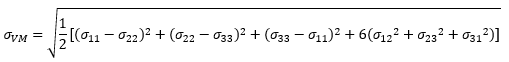The Von-Mises equation written above characterizes a 3d stress state that’s comprised of 6 different component stresses written in indicial notation. If a generalized stress state was written in matrix form, the stress tensor has 9 components, but due to isotropic elasticity, the shear stresses are equivalent (symmetric stress tensor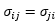).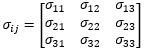Regardless of the state of stress (for a symmetric stress tensor), it’s always possible to orient a set of normal stresses “Principal Stresses” so that the shear stress components are zero. These principal stresses can be found by assuming that λ represents the unknown magnitude of the principal stresses oriented with no component of shear stress. Combined with, I, the identity matrix, the principal stresses can be found by solving: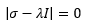For a given set of nine stress components, the solution to the equation above is a cubic equation for the unknown magnitude λ (the roots to det||=0), with three real roots for λ (σ1, σ2, and σ3 “principal stresses”). The values of Principal stresses do not depend upon the coordinate system in which the component stress was initially given. Therefore, they are “invariant” of the state of stress (their values do not change with respect to their orientation), and when the determinant of the equation above is expanded, the cubic equation takes the form of: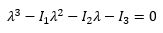If the axes of reference are chosen to coincide with the principal axes of stress, the scalar invariants I1, I2, I3 can be defined as the following expressions: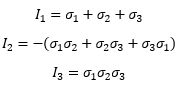The physical interpretation of the invariants are:

I1 is related to the hydrostatic component of the stress tensor.

I2 is related to the deviatoric parts of stress tensor.

I3 is the determinant of σij which is more difficult to describe using a physical interpretation, but if related to the deformation gradient rather than the stress tensor, this quantity would be associated with the volume ratio (VFinal/VOriginal, the ratio of deformed volume to initial volume), which is 1 for pure incompressible materials like rubber. A couple blogs on this topic are here: Determining Compressibility Properties for Hyperelastic FEA Models and Dealing With Incompressibility.

These invariants have played a prominent role in the development of constitutive equations that are used to model isotropic materials and the formulation of yield conditions under combined stress in theories of plasticity. Additionally, they are also rooted into material failure models that account for various modes of distortion.

One approach to account for the stress state while plastic straining is occurring is to track the stress triaxiality factor, η, which is the ratio the hydrostatic stress (mean stress, “pressure”, σm) to the effective stress, σVM. The triaxiality factor shown below is written in terms of both the invariants and the principal stresses, which would be readily available from a FEA solution.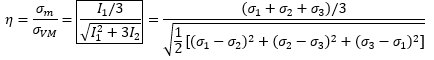It has been widely documented that moderate to high stress triaxiality (or increased hydrostatic stress, σm) leads to accelerated structural degradation under plastic deformation. As shown in Figure 2, at high stress triaxiality and dominating tensile loading, the plastic strain at failure is lower than if exposed to the effects of a compressive state. Therefore, the fracture strain is strongly dependent upon the level of stress triaxiality.

Figure 2: Plastic Failure Strain vs. Triaxiality Factor

## triax2.pngThe work of Snow, Morton, Pleins, and Keating outlines a strain-based acceptance criteria for energy-limited events based on the stress triaxiality factor. It has been used in the nuclear industry to determine the acceptability of designs and is incorporated into the ASME B&PV Code.

In metal cutting applications, a reduced strain to failure would be beneficial, as the material would liberate at a lower strain. This may lead a tool bit manufacturer to want to design the shape of their cutting tool to control the local triaxiality of the chip and produce a more efficient cut.

My next post will further expand upon the triaxiality factor and use it in conjunction with the Lode angle parameter to describe failure models. Until then, I’d be curious to hear about how you may be using stress triaxiality in your FEA simulations.

__________________________
References:

Introduction to the Mechanics of a Continuous Medium

LSTC LS-DYNA® Manual

Strain-Based Acceptance Criteria for Energy-Limited Events